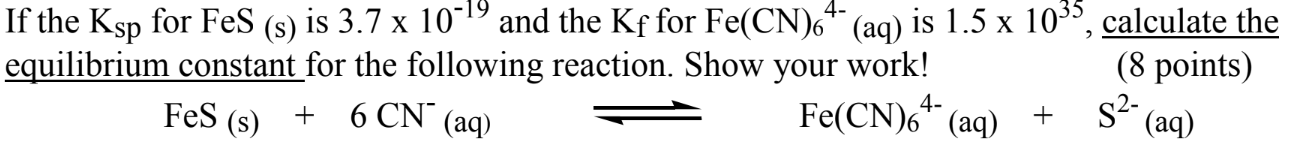# If the Ksp for FeS (s) is 3.7x10^(-19) and Kf for Fe(CN)6^(-4) is 1.5x10^35, calculate the equilibrium constant fo the following reaction. Show your work! FeS(s) + 6 CN^(-) (aq) ⇌ Fe(CN)6^(4-) (aq) + S^(2-) (aq)